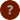whole word or phrase part of the word if you are searching for a compound word, note that it might appear in any of three ways, reflecting varied editorial practice: spaced ('house keeper'), solid ('housekeeper'), or hyphenated ('house-keeper') or use Advanced Search# Search results

## Search phrase: assume

### Plays

14 result(s).
PlayKey LineModern TextOriginal Text
HamletHam I.ii.244If it assume my noble father's person,If it assume my noble Fathers person,
HamletHam I.iv.72And there assume some other, horrible form,And there assumes some other horrible forme,
HamletHam II.ii.598T' assume a pleasing shape, yea, and perhapsT'assume a pleasing shape, yea and perhaps
HamletHam III.iv.161Assume a virtue, if you have it not.Assume a Vertue, if you haue it not,
Henry VH5 I.chorus.6Assume the port of Mars, and at his heels,Assume the Port of Mars, and at his heeles
King LearKL V.iii.185Into a madman's rags, t' assume a semblanceInto a mad-mans rags, t'assume a semblance
The Merchant of VeniceMV II.ix.51I will assume desert. Give me a key for this,I will assume desert; giue me a key for this,
The Merchant of VeniceMV III.ii.87And these assume but valour's excrementAnd these assume but valors excrement,
Much Ado About NothingMA I.i.300I will assume thy part in some disguiseI will assume thy part in some disguise,
Much Ado About NothingMA II.i.221answered her; my very visor began to assume life andanswered her: my very visor began to assume life, and
PericlesPer I.i.62Like a bold champion I assume the lists,Like a bold Champion I assume the Listes,
Timon of AthensTim IV.iii.219Rascals should have't. Do not assume my likeness.Rascals should haue't. Do not assume my likenesse.
Troilus and CressidaTC V.ii.148Without perdition, and loss assume all reasonWithout perdition, and losse assume all reason,
Twelfth NightTN V.i.232If spirits can assume both form and suitIf spirits can assume both forme and suite,

### Glossary

11 result(s).
 affect assume, display, put on, practise in an artificial way assume acquire, adopt, take on assume attain, achieve, reach assume undertake, enter, choose guess assume, surmise, suppose take assume, pretend, feign take undertake a role, assume a responsibility [for oneself] take assume a role, carry on take assume the burden of, undertake the study of [for oneself] undertake assume, take on, feign usurp assume, take on, adopt

### Thesaurus

10 result(s).
 assume affect assume usurp assume take assume guess assume undertake assume a burden take assume a responsibility take burden, assume a take responsibility, assume a take role, assume a take

1 result(s).

### Words Families

3 result(s).
Word FamilyWord Family GroupWords
ASSUMEBASICassume v, assumption n
ASSUMESTATEself-assumption n
SHAKESPEARE'S WORDS © 2020 DAVID CRYSTAL & BEN CRYSTAL